## Nexus.cqu.edu.au

CHEM11041 Chemistry for the Life Sciences
Assessment item 2 (weighting 10%)
Due: 11th May, 2012

Please provide responses to the following questions using the Optical Mark Reader (OMR)
form that will be supplied by your lecturers. You must follow the instruction that
accompany the OMR form.

You must attach a signed coversheet to your assessment item. The coversheet should be

Distance students should submit their assessment item through CQUCentral. The item
must be posted no later than the due date.

On-campus students should submit their assessment item in the designated assignment
box at their campus of study.

The completed OMR form should not be stapled to the coversheet or bent in any manner.
It is best submitted between two pieces of cardboard.

1. Which of the following is an example of a physical change?
2. The compound, 1-butanethiol is one of the compounds that gives skunks their distinctive odor. The compound freezes at -115.7°C and boils at 98.5°C. What is its phase at 37°C, the normal body temperature of humans? 3. Choose the correct response that satisfies the following statement. The electronic shell having principal quantum number, n = 3 contains ________ subshells, ________ orbitals, and up to ________ electrons 4. What is the formula of a compound formed by the combination of the ions M and X ? 5. Which statement below regarding balanced chemical equations is not true?
a) The number of each kind of atoms must be the same on each side. b) Coefficients are used in front of formulas to balance the equation. c) Subscripts may be changed to make an equation simpler to balance. d) When no coefficient is written in front of a formula, the number "one" is assumed. e) Reactants are written to the left of the arrow. 6. Which reaction is an example of both a precipitation and a neutralisation?
a) 2 H PO (aq) + 3 Ca(OH) (aq) → Ca (PO ) (aq) + 6 H O (l) b) FeCl (aq) + 3KOH (aq) → Fe(OH) (s) + 3 KCl (aq) c) (NH4)2CO3(s) → 2 NH (g) + CO (g) + H O (l) d) H SO (aq) + Ba(OH) (aq) → BaSO (s) + 2 H O (l) e) 2 C (s) + O (g) → 2 CO (g) 7. How many molecules are present in 4.25 mol of CCl ? 8. How many moles of NaHCO are present in a 2.00 g sample? 9. In the reaction shown below, what is the mole ratio that would be used to determine the
number of moles of H that would be produced when 3.5 moles of AlCl are produced?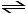10. Which of the following statements concerning free energy and spontaneity is not true?
If the value of ΔG for a reaction is 150 kJmol-1, the value of ΔG for the reverse reaction will be -150 kJmol-1. b) If the value of ΔG for a reaction is negative, the reaction is said to be spontaneous. A reaction which is not spontaneous at low temperature can become spontaneous at higher temperatures. d) Enthalpy and entropy are of equal importance in determining the spontaneity of a The speed of a reaction is not influenced by its spontaneity. 11. Which of the following statements describes how a catalyst works? 12. Consider the following reaction: 2 SO (g) + O (g) with equilibrium constant, K = 4.8 ×10 27 at 250C. Which statement about this system is not true?
a) At equilibrium SO is the predominant substance. b) Heating the system will cause breakdown of SO . c) Adding SO will cause an increase in the amount of SO . d) Removing O will cause an increase in the amount of SO . e) The large numerical value of K means that the reaction essentially goes to completion. 13. How many moles of air are present in a person’s lungs if they occupy a volume of 5.0L at 37oC and 1.0 atm pressure? Make sure you use the appropriate value for R to ensure that your calculation is dimensionally correct. 14. Which of the following statements is the best description of hydrogen bonding? a) The unique chemical bonds that form between hydrogen and any other atom in the same b) The association between hydrogen of one molecule and a region of another molecule which has become negative due to temporary shifts in electron density hydrogen bonds. c) The polarity associated with a bond between hydrogen and a small electronegative atom d) The association between a hydrogen atom which is somewhat positive and an atom of O, e) The temporary attraction between hydrogen atoms on different molecules resulting from 15. Which of the following molecules cannot form hydrogen bonds? 16. The amount of energy involved in melting a substance without changing its temperature is
17. What is the minimum number of components that must be present in a solution? 18. Which of the following solutions is the least concentrated? 19. What is the molarity of a solution prepared by dissolving 1.25 mol of AgNO in 625 mL of 20. What volume of 16 M NH is needed to prepare 2.00 L of a 2.00 M solution? 21. The movement of a solvent across a semipermeable membrane because of concentration22. Hydrogen cyanide, HCN, is a weak acid. Which of the following equations best represents its 23. Which one of the following represents a basic solution? 24. Given that the ion product constant for water, Kw=1.01 x 10-14 , what is the [OH ] if the 25. What is the hydrogen ion concentration in a solution that has a pH = 2.34?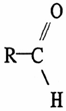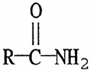26. The pH of a 250.0 mL sample of a buffer solution is 9.85. If 1.0 mL of 6 M HCl is added to the buffer solution the pH of the resulting mixture will be closest to: 27. Which form of radiation is generally considered the most dangerous? 28. Which of the following molecules can be classified as a hydrocarbon? 29. Which of the following represents the functional group of an ester?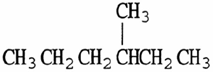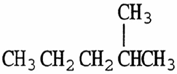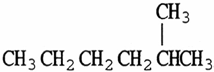30. Two or more compounds with the same molecular formula but with the atoms connected 31. Which of the following is an isomer of the molecule shown?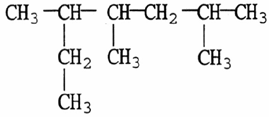32. The various shapes of an organic molecule are referred to as 33. What is the IUPAC name of the following compound ? 34. The name of the following molecule is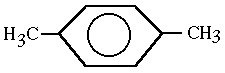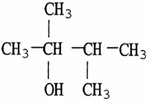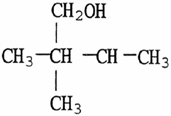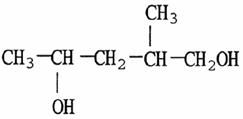35. Using systematic names, the structure shown could be called 36. All of the following are common reactions of benzene except 38. Treatment of CH CH OH with an excess amount of oxidising agent will produce
39. What is the product of the oxidation of a secondary alcohol? 40. Organic compounds that are sulfur analogs of alcohols are referred to as

Source: http://nexus.cqu.edu.au/assignmentview/displayDocument/1922/Assessment+item+2.pdf

### Norlevo patient info

Norlevo Pat Info_Norlevo patient Info 2013/07/12 9:19 AM Page 1 PASIËNT INLIGTINGSBROSJURE Lees hierdie hele voubiljet noukeurig voordat u hierdie medisyne neem. Hierdie medisyne is beskikbaar sonder ’n voorskrif vanaf u dokter, vir u om ’n toestand te behandel. Nieteenstaande moet u nog steeds NORLEVO versigtig gebruik om die beste resultate te verkry. • Hou hierdie voubiljet. U mag dit

### Publicationsupdate.hmi.0809

ORIGINAL, PEER REVIEWED ARTICLES Haddad, J., Jr, Angel, M.F., Abramson, M.: Reduction of Hematoma-Induced Flap Necrosis by Deferoxamine. Surgical Forum, Volume XXXVII, 1986:564-565. Angel, M.F., Haddad, J., Jr, Abramson, M.: A Free Radical Scavenger Reduces Hematoma-Induced Flap Necrosis in Fischer Rats. Otolaryngol Head Neck Surg, 1987; 96:96-98. Haddad, J., Jr., Isaacson, G., Respler, D.,